Search IntMath
Close

450+ Math Lessons written by Math Professors and Teachers

5 Million+ Students Helped Each Year

1200+ Articles Written by Math Educators and Enthusiasts

Simplifying and Teaching Math for Over 23 Years

# 4a. Volume of Solid of Revolution by Integration (Disk method)

by M. Bourne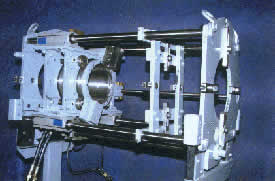A lathe

Many solid objects, especially those made on a lathe, have a circular cross-section and curved sides. On this page, we see how to find the volume of such objects using integration.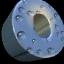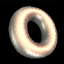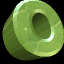Objects made on a lathe ...

### Applications

Don't miss the winecask and watermelon applications in this section.

Consider the area bounded by the straight line y = 3x, the x-axis, and x = 1:

The graph of y=3x, with the area under the "curve" between x=0 to x=1 shaded.

When the shaded area is rotated 360° about the x-axis, a volume is generated.

The resulting solid is a cone:

Area under the curve y=3x from x=0 to x=1 rotated around the x-axis, showing a typical disk.

## Disk Method for Finding Volumes

To find this volume, we could take slices (the dark green disk shown above is a typical slice), each dx wide and radius y:

The typical disk shown with its dimensions, radius = y and "height" = dx.

The volume of a cylinder is given by:

V = πr^2h

Because "radius" = r = y and each disk is dx high, we notice that the volume of each slice is:

V = πy^2\ dx

Adding the volumes of the disks (with infinitely small dx), we obtain the formula:

V=pi int_a^b y^2dx  which means   V=pi int_a^b {f(x)}^2dx

where:

y = f(x) is the equation of the curve whose area is being rotated

a and b are the limits of the area being rotated

dx shows that the area is being rotated about the x-axis

NOTE: On this page we use the disk method and washer method (where we cut the shape into circular slices) only, and meet the Shell Method next).

Applying the formula V=pi int_a^b y^2dx to the earlier example, we have:

"Vol" =pi int_a^b y^2dx

=pi int_0^1(3x)^2dx

=pi int_0^1 9x^2dx

=pi[3x^3]_0^1

=pi-pi

=3pi\ "unit"^3

CHECK: Does the method work? We can find the volume of the cone using

"Vol"=(pi r^2h)/3

 = (pi(3)^2(1))/3

=(9pi)/3

=3pi\ "unit"^3 (Checks OK.)

### Example 2

Find the volume if the area bounded by the curve y = x^3+ 1, the x-axis and the limits of x = 0 and x = 3 is rotated around the x-axis.

This is the region as described, under a cubic curve.

Area bounded by y = x^3+1, x=0 and x=3.

When the shaded area is rotated 360° about the x-axis, we observe that a volume is generated:

Area under the curve y=x^3+1 from x=0 to x=3 rotated around the x-axis, showing a typical disk.

Applying the formula for the solid of revolution, we get

V=pi int_a^b y^2 dx

=pi int_0^3(x^3+1)^2 dx

=pi int_0^3(x^6+2x^3+1) dx

=pi [(x^7)/(7)+(x^4)/(2)+x]_0^3

=pi(|355.93|-|0|)

=1118.2\ text[units]^3

## Volume by Rotating the Area Enclosed Between 2 Curves

If we have 2 curves y_2 and y_1 that enclose some area and we rotate that area around the x-axis, then the volume of the solid formed is given by:

"Volume"=pi int_a^b[(y_2)^2-(y_1)^2]dx

In the following general graph, y_2 is above y_1. The lower and upper limits for the region to be rotated are indicated by the vertical lines at x = a and x = b.

y_2
y_1

Area bounded by the curves y_1 and y_2, & the lines x=a and x=b.

When we rotate such a shape around an axis, and take slices, the result is a washer shape (with a round hole in the middle).

### Example 3

A cup-like object is made by rotating the area between y = 2x^2 and y = x + 1 with x ≥ 0 around the x-axis. Find the volume of the material needed to make the cup. Units are "cm".

We sketch the upper and lower bounding curves:

Area bounded by y = 2x^2 (the bottom curve), y = x+1 (the line above), and x = 0, showing a typical rectangle.

The lower limit of integration is x = 0 (since the question says x ≥ 0).

Next, we need to find where the curves intersect so we know the upper limit of integration.

Equating the 2 expressions and solving:

2x2 = x + 1

2x2x − 1 = 0

(2x + 1)(x − 1) = 0

x = 1 (since we only need to consider x ≥ 0. This is consistent with what we see in the graph above.)

So with y2 = x + 1 and y1 = 2x2, the volume required is given by:

text[Volume]=pi int_0^1 [(x+1)^2-(2x^2)^2]dx

=pi int_0^1 [(x^2+2x+1)-(4x^4)] dx

=pi [(x^3)/(3)+x^2+x-(4x^5)/(5)]_0^1

=pi[(1/3 + 1+1 - 4/5)-(0)]

=pi[(5+30-12)/(15)]

=(23pi)/(15)

=4.817\ text[cm]^3

Here's an illustration of the volume we have found. A typical "washer" with outer radius y2 = x + 1 and inner radius y1 = 2x2 is shown.

The cup resulting from rotating the area bounded by y = 2x^2, y = x+1, and x = 0 about the x-axis.

## Rotation around the y-axis

When the shaded area is rotated 360° about the y-axis, the volume that is generated can be found by:

V=pi int_c^d x^2dy  which means  V=pi int_c^d {f(y)}^2dy

where:

x =f(y) is the equation of the curve expressed in terms of y

c and d are the upper and lower y limits of the area being rotated

dy shows that the area is being rotated about the y-axis

### Example 4

Find the volume of the solid of revolution generated by rotating the curve y = x^3 between y = 0 and y = 4 about the y-axis.

Here is the region we need to rotate:

The graph of the area bounded by y=x^3, x=0 and y=4.

And here is the volume generated when we rotate the region around the y-axis:

The volume generated when revolving the curve bounded by y=x^3, x=0 and y=4 around the y-axis.

We first must express x in terms of y, so that we can apply the volume of solid of revolution formula.

If y = x3 then x = y1/3

The formula requires x2, and on squaring we have x2 = y2/3

text[Vol] = pi int_c^d x^2 dy

=pi int_0^4 y^[2//3] dy

=pi [(3y^[5//3])/(5)]_0^4

=(3pi)/(5)[y^[5//3]]_0^4

=(3pi)/(5)[10.079-0]

=19.0\ text[units]^3

## Exercises

Find the volume generated by the areas bounded by the given curves if they are revolved about the given axis:

(1) The straight line y = x, between y = 0 and x = 2, revolved about the x-axis.

This is the area to be rotated around the x-axis:

The graph of y=x, with the area under the "curve" between x=0 to x=2 shaded.

Hence, the volume generated can be found using the formula for volume of solid of revolution:

"Vol" = pi int_a^b y^2 dx

=pi int_0^2 (x)^2 dx

= pi[(x^3)/(3)]_0^2

=pi[(8)/(3)]-pi

=8/3 pi\ text[units]^3

~~8.378\ text[units]^3

(2) The curve y = 2x − x^2 bounded by y = 0, revolved about the x-axis.

The graph of y=2x-x^2, with the area under the curve between x=0 to x=2 shaded.

The line y=0 simply means the x-axis.

From the diagram, we can see that the limits of the bounded area are x = 0 and x = 2.

The volume generated is:

"Vol"=pi int_a^b y^2 dx

=pi int_0^2 (2x-x^2)^2 dx

=pi int_0^2 (4x^2-4x^3+x^4) dx

=pi [(4x^3)/(3) - (4x^4)/(4) + (x^5)/(5)]_0^2

=pi [32/3 - 16 + 32/5] - pi

=(16)/(15)pi\ text[units]^3

~~3.351\ text[units]^3

(3) The curve y^2 = x, bounded by y = 4 and x = 0, revolved about the y-axis.

The graph of the area bounded by y^2=x, y=4 and x=0.

Since x=y^2, we have x^2=y^4.

Hence, the volume generated is:

"Vol"= pi int_c^d x^2 dy

=pi int_0^4 y^4 dy

=pi [(y^5)/(5)]_0^4

=pi[(1024)/(5)] - pi

=(1024)/(5) pi\ text[units]^3

~~643.40\ text[units]^3

(4) The curve x^2 + 4y^2 = 4 in quadrant I, revolved around the y-axis.

We recognise that this is an ellipse. The question tells us the area of interest is in the first quadrant only.

The ellipse x2 + 4y2 = 4, showing the portion bounded by the curve, x = 0, x = 2 and the x-axis.

From the diagram, we can see that the limits of the bounded area are y = 0 and y = 1.

Hence the volume generated is:

"Vol"=pi int_c^d x^2 dy

=pi int_0^1 (4-4y^2) dy

=pi[4y-(4y^3)/(3)]_0^1

=pi[4-4/3] - pi

=(8pi)/(3)\ text[units]^3

~~8.378\ text[units]^3

## Applications

### 1. Volume of a wine cask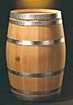A wine cask has a radius at the top of 30 cm and a radius at the middle of 40 cm. The height of the cask is 1 m. What is the volume of the cask (in L), assuming that the shape of the sides is parabolic?

We will lay the cask on its side to make the algebra easier:

Parabola with vertex at (0, 40) and passing through (50, 30).

We need to find the equation of a parabola with vertex at (0, 40) and passing through (50, 30).

We use the formula:

(x h)2 = 4a(y k)

Now (h, k) is (0, 40) so we have: x2 = 4a(y − 40) and the parabola passes through (50, 30), so

(50)2 = 4a(30 − 40)

2500 = 4a(−10) and this gives 4a = −250

So the equation of the side of the barrel is

x2 = -250(y − 40), that is,

y=-(x^2)/(250)+40

We need to find the volume of the cask which is generated when we rotate this parabola between x = -50 and x = 50 around the x-axis.

Parabolic area rotated around the x-axis producing our wine cask.

We now apply the formula for the volume of a solid of revolution:

"Vol"=pi int_a^by^2\ dx

=pi int_-50^50(-(x^2)/(250)+40)^2 dx

=pi int_-50^50((x^4)/(62500) - (80x^2)/(250)+1600) dx

=pi[(x^5)/(312500)-(80x^3)/(750)+1600x]_-50^50

Now, since

(−50)5 = −505,

(−50)3 = −503, and

(−50) = −50,

we can reduce the amount of writing somewhat and put:

text[Vol] = 2pi[((50)^5)/(312500)-(80(50)^3)/(750)+1600(50)]

=425162\ text[cm]^3

=425.2\ text[L]

So the wine cask will hold 425.2\ "L".

### 2. Volume of a watermelon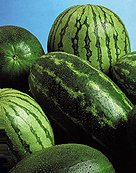A watermelon has an ellipsoidal shape with major axis 28 cm and minor axis 25 cm. Find its volume.

Historical Approach: Before calculus, one way of approximating the volume would be to slice the watermelon (say in 2 cm thick slices) and add up the volumes of each slice using V = πr^2h.

Interestingly, Archimedes (the one who famously jumped out of his bath and ran down the street shouting "Eureka! I've got it") used this approach to find volumes of spheres around 200 BC. The technique was almost forgotten until the early 1700s when calculus was developed by Newton and Leibniz.

We see how to do the problem using both approaches.

Volume using historical method: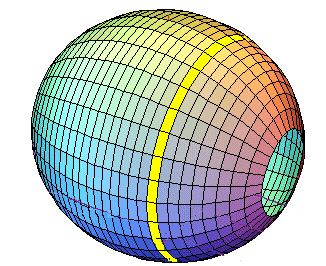Because the melon is symmetrical, we can work out the volume of one half of the melon, and then double our answer.

The radii for the slices for one half of a particular watermelon are found from measurement to be:

0, 6.4, 8.7, 10.3, 11.3, 12.0, 12.4, 12.5.

The approximate volume for one half of the melon using slices 2 cm thick would be:

"V"_[text[half]]=pi times [6.4^2 + 8.7^2+10.3^2+11.3^2 +12.0^2 +12.4^2 {:+12.5^2] times 2

= pi times 8040.44 times 2

=5054.4

So the volume for the whole watermelon is about

5054.4 × 2 = 10109\ "cm"^3= 10.1\ "L".

In the following question, we see how to find the "exact" value using the volume of solid of revolution formula.

"Exact" Volume (using Integration):

We are told the melon is an ellipsoid. We need to find the equation of the cross-sectional ellipse with major axis 28 cm and minor axis 25 cm.

We use the formula (from the section on ellipses):

(x^2)/(a^2)+(y^2)/(b^2)=1

where a is half the length of the major axis and b is half the length of the minor axis.

For the volume formula, we will need the expression for y2 and it is easier to solve for this now (before substituting our a and b).

(x^2)/(a^2)+(y^2)/(b^2)=1

b^2x^2+a^2y^2=a^2b^2

a^2y^2=a^2b^2-b^2x^2=b^2(a^2-x^2)

y^2=(b^2)/(a^2)(a^2-x^2)

Since a = 14 and b = 12.5, we have:

y^2=(12.5^2)/(14^2)(14^2-x^2)

=0.797(196-x^2)

NOTE: The a and b that we are using for the ellipse formula are not the same a and b we use in the integration step. They are completely different parts of the problem.

Using this, we can now find the volume using integration. (Once again we find the volume for half and then double it at the end).

text[V]_[text[half]]=pi int_0^14y^2 dx

=pi int_0^14 0.797(196-x^2)dx

=0.797pi int_0^14(196-x^2)dx

=2.504[196x-(x^3)/(3)]_0^14

=2.504[196(14)-(14^3)/(3)]

=2.504 times 1829.33

=4580.65\ text[cm]^3

So the watermelon's total volume is 2 × 4580.65 = 9161\ "cm"^3 or 9.161\ "L". This is about the same as what we got by slicing the watermelon and adding the volume of the slices.

## Problem SolverThis tool combines the power of mathematical computation engine that excels at solving mathematical formulas with the power of GPT large language models to parse and generate natural language. This creates math problem solver thats more accurate than ChatGPT, more flexible than a calculator, and faster answers than a human tutor. Learn More.